Electricity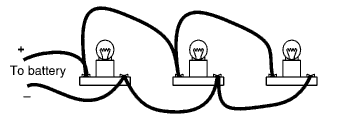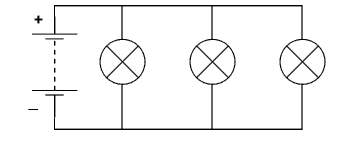More On Circuits
Is this set up a series
circuit or a parallel circuit ?
An accurate diagram of
the circuit in Figure A.
Figure A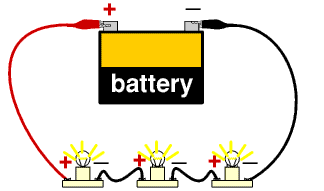Figure B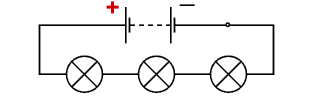Here's another circuit.
Is it series or parallel?
An accurate diagram of
the circuit in Figure B.
Look closely at Figure B. What happens when the wire is disconnected from the negative terminal? When the current is broken, look what happens in the diagram of the circuit.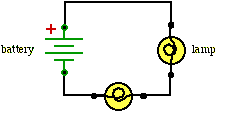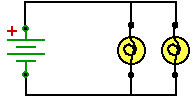Here are two more circuits.
Which one is a series and which one is parallel?
Diagram A
Diagram B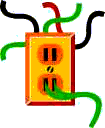If you wish to try a few more on-line circuit questions then click here.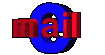bj
bj's MST Homepage
Teacher Resources
BACK
NEXT
Electric Circuits
series and parallel
magnets and
electromagnetism